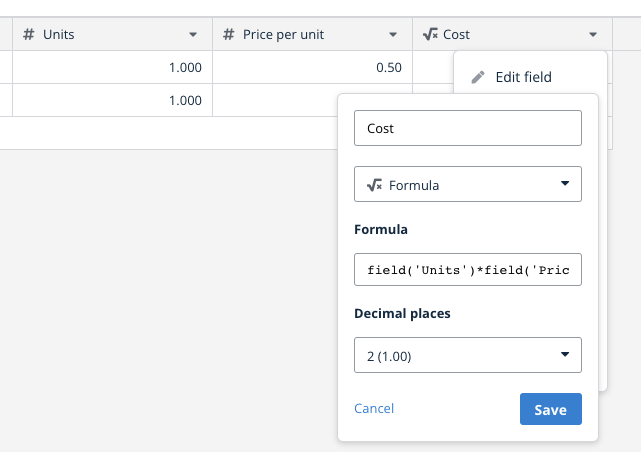Hello everyone! I’m new to Baserow and I have a question regarding data manipulation. To better illustrate my question, I’ll use the Recipe Book template provided by the platform. Taking the example of row 2 in the Dishes table, a Cheese Burger has a list of ingredients associated with its composition, and in the “Cost (all ingredients)” field, the unit cost of each ingredient is summed up. My question is, how can I create a new field with the quantities used for each ingredient, in order to multiply the unit cost by the quantity (example: Mustard - Quantity: 30g x Cost: \$2 = \$0.60), and then sum up each multiplication to obtain the final cost of the recipe?

Hello @igormts ,

I think you need to structure your tables slightly differently. Here a screenshot of what I mean:

First of all you need a table where you link your dish with every single ingredient where you can define the quantity, the price per unit, and the total cost of for that specific ingredient for the dish.Then in the dishes table you can do a `sum(lookup('PerDishIngredient', 'Cost'))` to have the total cost for the dish.

davide

1 Like

Thank you for the feedback, David! I had a feeling I would have to do it that way, but I was hoping to find a more optimized approach because the system I’m developing will be multi-company and will consume a lot of table rows since each recipe has an average of 12 ingredients.

It might exists a more complicated way to solve the problem using fewer rows in the database, but is it really worth it?

Baserow can easily handle millions of rows, and simple data structures like this allow for flexibility and simplicity in the final application.

If my math is correct, 12 lines of ingredients per dish + 1 line for the dish means you need totals to 13 lines on average, which means you can store more than 200 dishes in the free plan.

And if you have more rows in the future, you can easily upgrade to one of the paid plans or just self-host Baserow to be unlimited in rows.

If you think there is something we lack in terms of functionality (that can help you build your use case more easily), feel free to submit your idea here: Feature Ideas - Baserow.

David, thank you again for your feedback! I followed your data modeling guidance, where I created a table to input the recipes, including a field that multiplies the unit cost by the quantity of each ingredient. I also created another table to calculate the final cost of the recipe. However, when I tried to sum the field that performed the multiplication of quantity by unit cost, I received an error message stating, “Error with formula: argument number 1 given to function sum was of type array but the only usable type for this argument is a list of number values obtained from a lookup or link row field reference.” I didn’t quite understand how to correct this error while maintaining the suggested data modeling. I apologize for so many questions; it’s just that everything is really new to me. Thank you!
Note: I couldn’t insert screen images because I’m a new user on the forum.

Hey @igormts, you should be able to upload a file nowHey @igormts,

no need to apologize, we’re here to help and formulas are quite an advanced concept.

So the thing is that the lookup function returns an array of ingredients costs even if there’s only one ingredient in the linked table field, so for `CustoTotal` I think you should try something like:

`field('Quantitade') * sum(lookup('mercadoriasPrePreparo', 'CustoUnitario'))`

So, I found a solution, don’t know if it’s the correct one, but it’s what worked for me, and I’m gonna share it in case someone else has the same question. Apparently, the sum formula doesn’t add up the values from a field when that field is linked to another table. For example, in the field where I multiply the quantity of ingredient X by the unit cost of the ingredient (which is in the ‘mercadorias’ table), when I tried to sum up the result of this multiplication, I would get an error saying that it wasn’t possible to sum up an array. So what I did was create another field next to the one that performed the multiplication of the quantity by the unit cost, using the sum formula, which gave me the same result but now without being linked to the ‘mercadorias’ table. Then, I was able to sum up these results to get the final cost of the recipe.

Step 1:

Step 2:

Step 3:

Thank you for the help!